# Inverse Trigonometric Functions

## Introduction to Inverse Trigonometric Functions

• We know from previous chapter, A function is invertable if it is one-one and onto both and we can make function invertible by restricting the domain and range of the function
• Now we know that trigonometric function are many one function,so it is not possible to define the inverse function in thet domain and range
• But we can restrict the domain and range of the function to make them invertible.
• In this page, we will check out the inverse of all the six trigonometric functions with their domain and range.

## Inverse of Sin function

• sin function is a many one function with Domain and range as given below
Domain= R
Range =[-1,1]
• if we restrict its domain to $[-\frac {\pi}{2},\frac {\pi}{2}]$ , then it becomes one-one and onto with the range [-1,1]
• This is also true if we restrict its domain to $[\frac {\pi}{2},\frac {3\pi}{2}]$, $[-\frac {3\pi}{2},\frac {-\pi}{2}]$,.. , then also it becomes one-one and onto with the range [-1,1]. So it is becoming invertible with different branches
• The branch $[-\frac {\pi}{2},\frac {\pi}{2}]$ is the called the Principal value branch
• We define inverse sin for this branch only
• So inverse sin function is defined as
f: [-1,1] -> $[-\frac {\pi}{2},\frac {\pi}{2}]$ $f(x) =sin^{-1}(x)$
• Also $sin (sin^{-1} x) =x$ where $x \in [-1,1]$ and $sin^{-1} (sin x) =x$ where $x \in [-\frac {\pi}{2},\frac {\pi}{2}]$
• Also $y=sin^{-1} x$ then $x= sin y$
• Sometimes the function $sin^{-1} x$ is written as arc sin (x)

### Graph of Inverse Sin function

• The graph of the sin function in the Domain $[-\frac {\pi}{2},\frac {\pi}{2}]$ is given by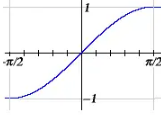• The graph of the inverse sin function can be obtained by interchanging the x and y axis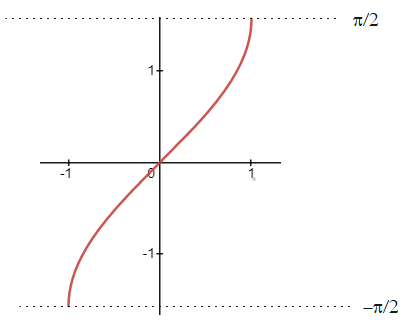## Inverse of Cos function

• cos function is a many one function with Domain and range as given below
Domain= R
Range =[-1,1]
• if we restrict its domain to $[0,\pi]$ , then it becomes one-one and onto with the range [-1,1]
• This is also true if we restrict its domain to $[\pi,2\pi]$, $[-\pi,0]$,.. , then also it becomes one-one and onto with the range [-1,1]. So it is becoming invertible with different branches
• The branch $[0,\pi]$ is the called the Principal value branch
• We define inverse cos for this branch only
• So inverse cos function is defined as
f: [-1,1] -> $[0,\pi]$ $f(x) =cos^{-1}(x)$
• Also $cos (cos^{-1} x) =x$ where $x \in [-1,1]$ and $cos^{-1} (cos x) =x$ where $x \in [0,\pi]$
• Also $y=cos^{-1} x$ then $x= cos y$
• Sometimes the function $cos^{-1} x$ is written as arc cos (x)

### Graph of Inverse cos function

• The graph of the cos function in the Domain $[0,\pi]$ is given by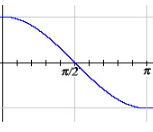• The graph of the inverse cos function can be obtained by interchanging the x and y axis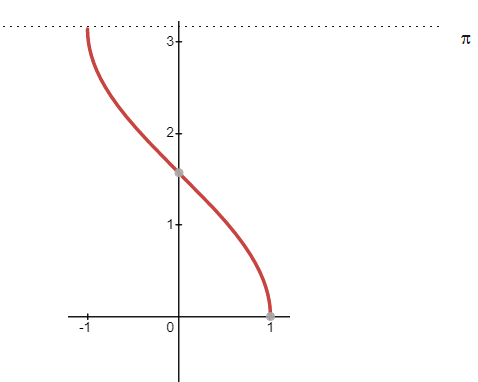## Inverse of Cosec function

• cosec function is a many one function with Domain and range as given below
Domain= [x:$x \in R$ and $x \neq n \pi$, $n \in Z$]
Range =[y:$|y| \geq 1$]
$f(x) =cosec x= \frac {1}{sin x}$
• if we restrict its domain to $[-\frac {\pi}{2},\frac {\pi}{2}] - {0}$ , then it becomes one-one and onto with the range R-(-1,1)
• This is also true if we restrict its domain to $[\frac {\pi}{2},\frac {3\pi}{2}] - {\pi}$, $[-\frac {3\pi}{2},\frac {-\pi}{2}] - {-\pi}$,.. , then also it becomes one-one and onto with the range R-(-1,1). So it is becoming invertible with different branches
• The branch $[-\frac {\pi}{2},\frac {\pi}{2}] -{0}$ is the called the Principal value branch
• We define inverse cosec for this branch only
• So inverse cos function is defined as
f: R-(-1,1) -> $[-\frac {\pi}{2},\frac {\pi}{2}] - {0}$ $f(x) =cosec^{-1}(x)$
• Also $cosec (cosec^{-1} x) =x$ where $x \in R-(-1,1)$ and $cosec^{-1} (cosec x) =x$ where $x \in [-\frac {\pi}{2},\frac {\pi}{2}] -{0}$
• Also $y=cosec^{-1} x$ then $x= cosec y$
• Sometimes the function $cosec^{-1} x$ is written as arc cosec (x)

### Graph of Inverse cosec function

• The graph of the cosec function in the Domain $[-\frac {\pi}{2},\frac {\pi}{2}] - {0}$ is given by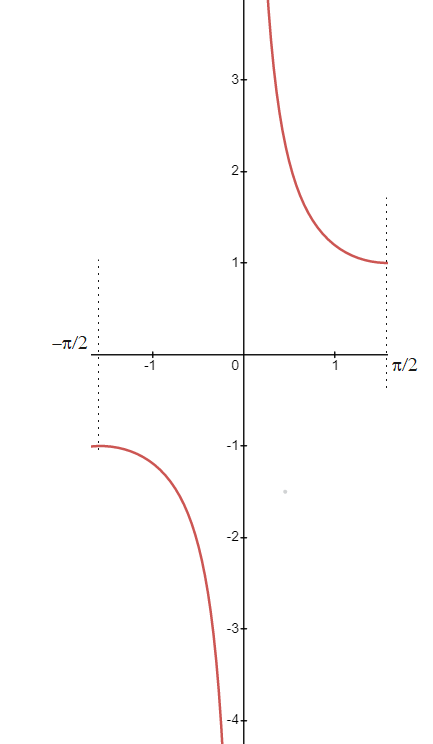• The graph of the inverse cosec function can be obtained by interchanging the x and y axis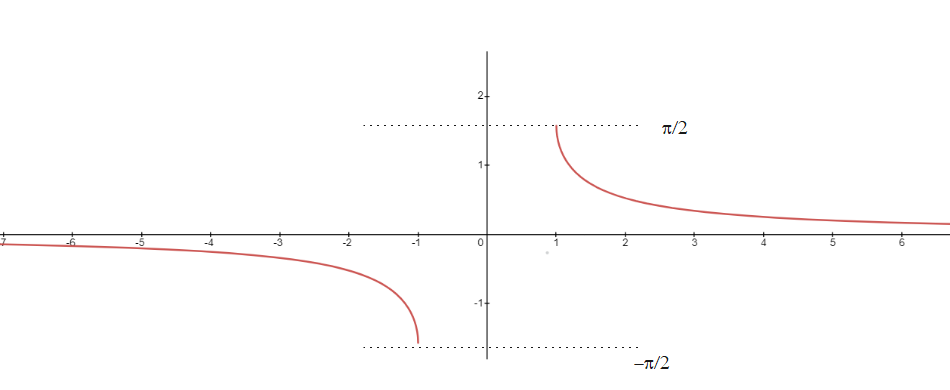## Inverse of sec function

• sec function is a many one function with Domain and range as given below
Domain= [x:$x \in R$ and $x \neq \frac {(2n+1)}{2} \pi$, $n \in Z$]
Range =[y:$|y| \geq 1$]
$f(x) =sec x= \frac {1}{sec x}$
• if we restrict its domain to $[0,\pi] - \frac {\pi}{2}$ , then it becomes one-one and onto with the range R-(-1,1)
• This is also true if we restrict its domain to $[\pi,2\pi] -\frac {3\pi}{2}$, $[-\pi,0] - \frac {-\pi}{2}$,.. , then also it becomes one-one and onto with the R-(-1,1). So it is becoming invertible with different branches
• The branch $[0,\pi] - \frac {\pi}{2}$ is the called the Principal value branch
• We define inverse sec for this branch only
• So inverse cos function is defined as
f: R-(-1,1) -> $[0,\pi] - \frac {\pi}{2}$, $f(x) =sec^{-1}(x)$
• Also $sec (sec^{-1} x) =x$ where $x \in R-(-1,1)$ and $sec^{-1} (sec x) =x$ where $[0,\pi] - \frac {\pi}{2}$
• Also $y=sec^{-1} x$ then $x= sec y$
• Sometimes the function $sec^{-1} x$ is written as arc sec (x)

### Graph of Inverse sec function

• The graph of the cos function in the Domain $[0,\pi]$ is given by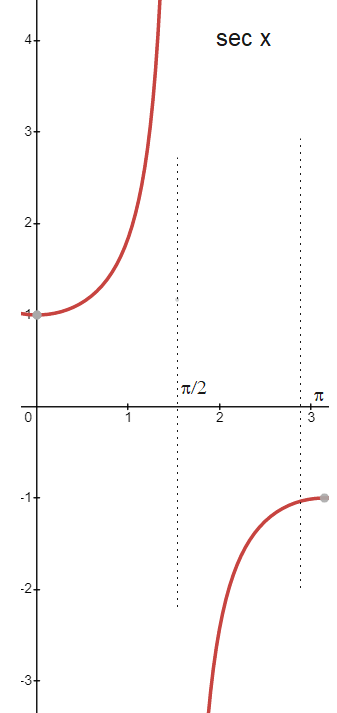• The graph of the inverse cos function can be obtained by interchanging the x and y axis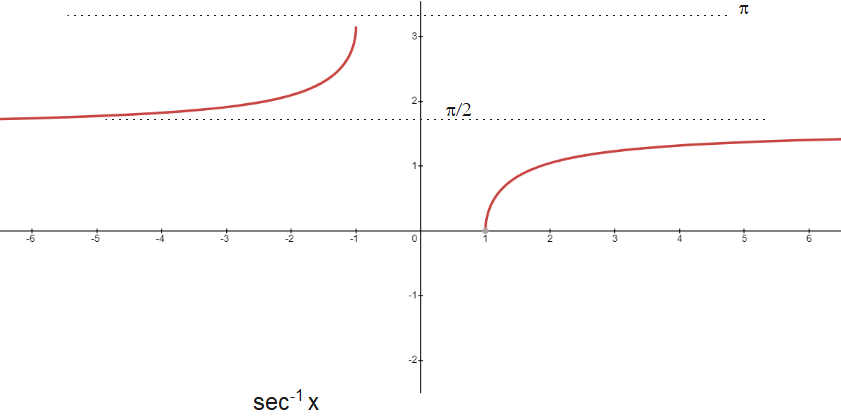## Inverse of tan function

• tan function is a many one function with Domain and range as given below
Domain= [x:$x \in R$ and $x \neq \frac {(2n+1}{2} \pi$, $n \in Z$]
Range =R
• if we restrict its domain to $(-\frac {\pi}{2},\frac {\pi}{2})$ , then it becomes one-one and onto with the range R
• This is also true if we restrict its domain to $(\frac {\pi}{2},\frac {3\pi}{2})$, $(-\frac {3\pi}{2},\frac {-\pi}{2})$,.. , then also it becomes one-one and onto with the range R. So it is becoming invertible with different branches
• The branch $(-\frac {\pi}{2},\frac {\pi}{2})$ is the called the Principal value branch
• We define inverse tan for this branch only
• So inverse sin function is defined as
f: R -> $(-\frac {\pi}{2},\frac {\pi}{2})$ $f(x) =tan^{-1}(x)$
• Also $tan (tan^{-1} x) =x$ where $x \in R$ and $tan^{-1} (tan x) =x$ where $x \in (-\frac {\pi}{2},\frac {\pi}{2})$
• Also $y=tan^{-1} x$ then $x= tan y$
• Sometimes the function $tan^{-1} x$ is written as arc tan (x)

### Graph of Inverse tan function

• The graph of the tan function in the Domain $(-\frac {\pi}{2},\frac {\pi}{2})$ is given by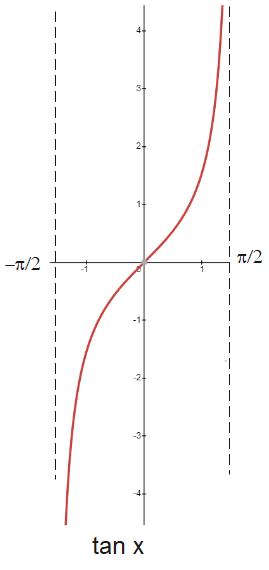• The graph of the inverse tan function can be obtained by interchanging the x and y axis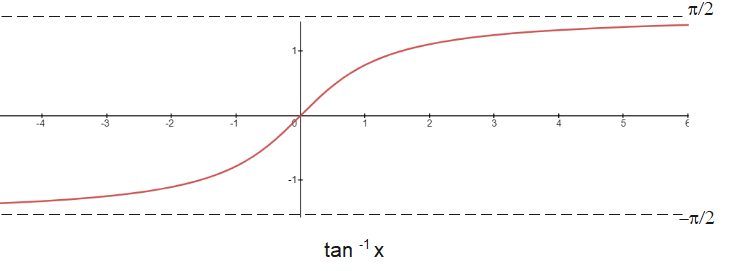## Inverse of cot function

• cot function is a many one function with Domain and range as given below
Domain= [x:$x \in R$ and $x \neq n\pi$, $n \in Z$]
Range =R
$cot(x) = \frac {1}{tan x}$
• if we restrict its domain to $(0,\pi)$ , then it becomes one-one and onto with the range R
• This is also true if we restrict its domain to $(\pi,2\pi)$, $(-\pi,0)$,.. , then also it becomes one-one and onto with the range R. So it is becoming invertible with different branches
• The branch $(0,\pi)$ is the called the Principal value branch
• We define inverse cot for this branch only
• So inverse cot function is defined as
f: R -> $(0,\pi)$ $f(x) =cot^{-1}(x)$
• Also $cot (cot^{-1} x) =x$ where $x \in R$ and $cot^{-1} (cot x) =x$ where $x \in (0,\pi)$
• Also $y=cot^{-1} x$ then $x= cot y$
• Sometimes the function $cot^{-1} x$ is written as arc cot (x)

### Graph of Inverse cot function

• The graph of the cot function in the Domain $(0,\pi)$ is given by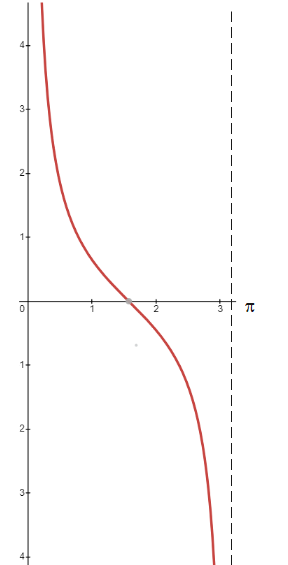• The graph of the inverse cot function can be obtained by interchanging the x and y axisGo back to Class 12 Main Page using below links